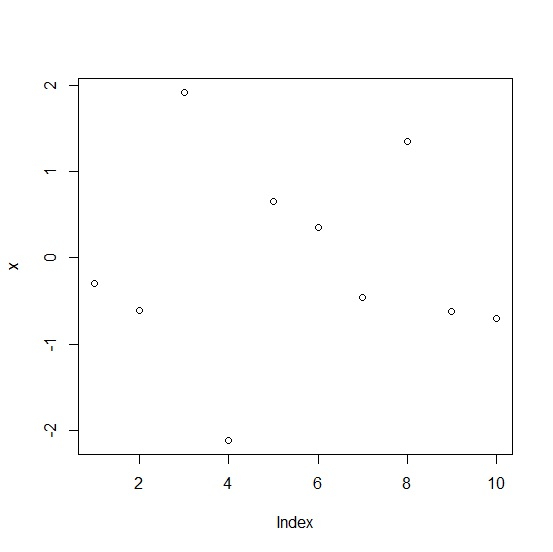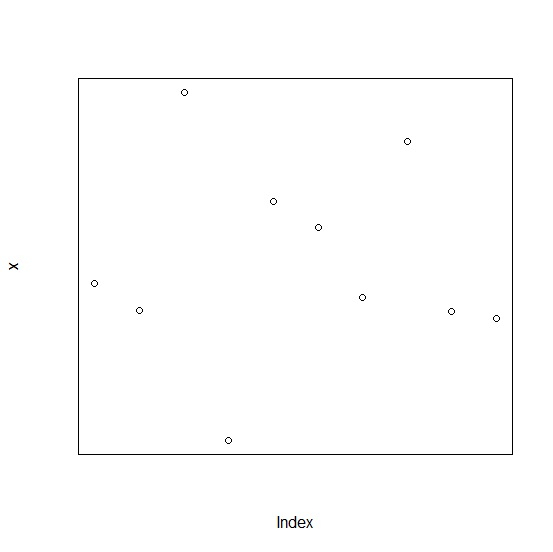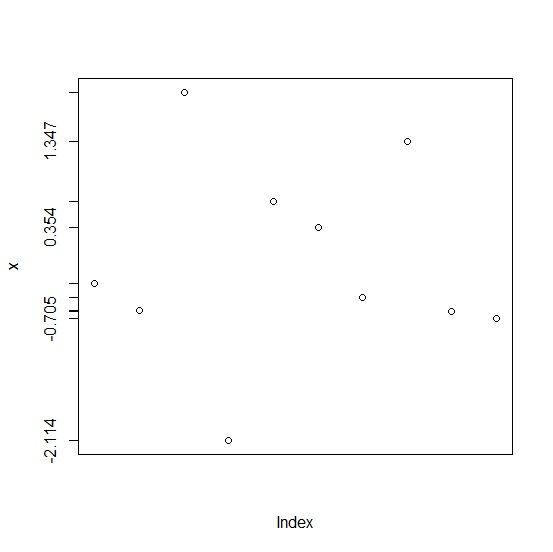# How to display Y-axis labels with more decimal places in R?

To display Y-axis labels with more decimal places, we would need to round the values of the vector or column for which we want to create the plot to the appropriate number of decimal places.

After that axis function will be used for creating the graph. Before doing all this, the graph of original values should be created without axes.

Check out the below example to understand how it works.

## Example

Following snippet creates a sample data frame −

x<-rnorm(10)
x

## Output

The following dataframe is created −

 -0.3002845 -0.6121883 1.9147725 -2.1135920 0.6575177 0.3540003
 -0.4564178 1.3474739 -0.6195008 -0.7050753

To display Y-axis labels with more decimal places in R, add the following code to the above snippet −

x<-rnorm(10)
plot(x)

## Output

If you execute all the above given snippets as a single program, it generates the following output −To display Y-axis labels with more decimal places in R, add the following code to the above snippet −

x<-rnorm(10)
plot(x,axes=FALSE,frame.plot=TRUE)

## Output

If you execute all the above given snippets as a single program, it generates the following output −To display Y-axis labels with more decimal places in R, add the following code to the above snippet −

x<-rnorm(10)
plot(x,axes=FALSE,frame.plot=TRUE)
Labels<-round(x,3)
axis(2,at=Labels,labels=Labels)

## Output

If you execute all the above given snippets as a single program, it generates the following output −